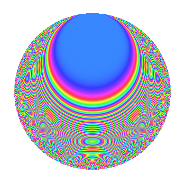# Properties

 Label 630.2.tLevel 630 Weight 2 Character orbit t Rep. character $$\chi_{630}(311,\cdot)$$ Character field $$\Q(\zeta_{6})$$ Dimension 64 Newforms 3 Sturm bound 288 Trace bound 1

# Learn more about

## Defining parameters

 Level: $$N$$ = $$630 = 2 \cdot 3^{2} \cdot 5 \cdot 7$$ Weight: $$k$$ = $$2$$ Character orbit: $$[\chi]$$ = 630.t (of order $$6$$ and degree $$2$$) Character conductor: $$\operatorname{cond}(\chi)$$ = $$63$$ Character field: $$\Q(\zeta_{6})$$ Newforms: $$3$$ Sturm bound: $$288$$ Trace bound: $$1$$ Distinguishing $$T_p$$: $$11$$

## Dimensions

The following table gives the dimensions of various subspaces of $$M_{2}(630, [\chi])$$.

Total New Old
Modular forms 304 64 240
Cusp forms 272 64 208
Eisenstein series 32 0 32

## Trace form

 $$64q$$ $$\mathstrut +\mathstrut 32q^{4}$$ $$\mathstrut -\mathstrut 4q^{7}$$ $$\mathstrut +\mathstrut 6q^{9}$$ $$\mathstrut +\mathstrut O(q^{10})$$ $$64q$$ $$\mathstrut +\mathstrut 32q^{4}$$ $$\mathstrut -\mathstrut 4q^{7}$$ $$\mathstrut +\mathstrut 6q^{9}$$ $$\mathstrut +\mathstrut 12q^{13}$$ $$\mathstrut +\mathstrut 6q^{14}$$ $$\mathstrut +\mathstrut 4q^{15}$$ $$\mathstrut -\mathstrut 32q^{16}$$ $$\mathstrut -\mathstrut 8q^{18}$$ $$\mathstrut +\mathstrut 22q^{21}$$ $$\mathstrut +\mathstrut 6q^{24}$$ $$\mathstrut +\mathstrut 64q^{25}$$ $$\mathstrut +\mathstrut 24q^{26}$$ $$\mathstrut +\mathstrut 36q^{27}$$ $$\mathstrut +\mathstrut 4q^{28}$$ $$\mathstrut -\mathstrut 6q^{29}$$ $$\mathstrut +\mathstrut 4q^{30}$$ $$\mathstrut -\mathstrut 12q^{31}$$ $$\mathstrut +\mathstrut 24q^{33}$$ $$\mathstrut +\mathstrut 4q^{37}$$ $$\mathstrut +\mathstrut 36q^{39}$$ $$\mathstrut -\mathstrut 6q^{41}$$ $$\mathstrut -\mathstrut 32q^{42}$$ $$\mathstrut +\mathstrut 4q^{43}$$ $$\mathstrut -\mathstrut 12q^{44}$$ $$\mathstrut +\mathstrut 6q^{45}$$ $$\mathstrut -\mathstrut 6q^{46}$$ $$\mathstrut +\mathstrut 36q^{47}$$ $$\mathstrut -\mathstrut 8q^{49}$$ $$\mathstrut +\mathstrut 8q^{51}$$ $$\mathstrut -\mathstrut 72q^{53}$$ $$\mathstrut -\mathstrut 36q^{54}$$ $$\mathstrut -\mathstrut 12q^{57}$$ $$\mathstrut -\mathstrut 60q^{59}$$ $$\mathstrut +\mathstrut 8q^{60}$$ $$\mathstrut +\mathstrut 102q^{61}$$ $$\mathstrut -\mathstrut 80q^{63}$$ $$\mathstrut -\mathstrut 64q^{64}$$ $$\mathstrut -\mathstrut 12q^{65}$$ $$\mathstrut -\mathstrut 48q^{66}$$ $$\mathstrut -\mathstrut 28q^{67}$$ $$\mathstrut -\mathstrut 6q^{70}$$ $$\mathstrut +\mathstrut 8q^{72}$$ $$\mathstrut -\mathstrut 12q^{77}$$ $$\mathstrut +\mathstrut 16q^{78}$$ $$\mathstrut +\mathstrut 8q^{79}$$ $$\mathstrut -\mathstrut 38q^{81}$$ $$\mathstrut +\mathstrut 14q^{84}$$ $$\mathstrut +\mathstrut 12q^{85}$$ $$\mathstrut +\mathstrut 96q^{87}$$ $$\mathstrut +\mathstrut 6q^{89}$$ $$\mathstrut +\mathstrut 24q^{91}$$ $$\mathstrut -\mathstrut 36q^{92}$$ $$\mathstrut +\mathstrut 8q^{93}$$ $$\mathstrut +\mathstrut 6q^{96}$$ $$\mathstrut +\mathstrut 12q^{97}$$ $$\mathstrut -\mathstrut 48q^{98}$$ $$\mathstrut -\mathstrut 48q^{99}$$ $$\mathstrut +\mathstrut O(q^{100})$$

## Decomposition of $$S_{2}^{\mathrm{new}}(630, [\chi])$$ into irreducible Hecke orbits

Label Dim. $$A$$ Field CM Traces $q$-expansion
$$a_2$$ $$a_3$$ $$a_5$$ $$a_7$$
630.2.t.a $$4$$ $$5.031$$ $$\Q(\zeta_{12})$$ None $$0$$ $$0$$ $$-4$$ $$2$$ $$q-\zeta_{12}q^{2}+(-\zeta_{12}+2\zeta_{12}^{3})q^{3}+\zeta_{12}^{2}q^{4}+\cdots$$
630.2.t.b $$28$$ $$5.031$$ None $$0$$ $$-2$$ $$-28$$ $$-4$$
630.2.t.c $$32$$ $$5.031$$ None $$0$$ $$2$$ $$32$$ $$-2$$

## Decomposition of $$S_{2}^{\mathrm{old}}(630, [\chi])$$ into lower level spaces

$$S_{2}^{\mathrm{old}}(630, [\chi]) \cong$$ $$S_{2}^{\mathrm{new}}(63, [\chi])$$$$^{\oplus 4}$$$$\oplus$$$$S_{2}^{\mathrm{new}}(126, [\chi])$$$$^{\oplus 2}$$$$\oplus$$$$S_{2}^{\mathrm{new}}(315, [\chi])$$$$^{\oplus 2}$$